#### 相似文章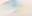# 构建自动运行的 EA（第 03 部分）：新函数

MetaTrader 5交易 | 13 三月 2023, 10:001 5700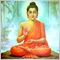### 为什么我们需要新函数？

• 其中一人将发送订单，以市价买入或卖出;
• 另一个则允许我们更改订单价格。

• 高波动时刻，订单“跳价”的可能（在 OCO 订单的情况下）。 排除 STOP LIMIT 订单，订单簿中的订单永远不会跳价，它们可以在所需点位之外触发，但它们永远不会跳价
• 连接问题，EA 可能在一段时间内无法访问服务器时；
• 涉及流动性的问题，订单可以坐在那里，等待执行，但交易量不足以实际执行;
• 最糟糕的是：EA 可以开始随机执行订单。

### 实现所需函数

```inline void CommonData(const ENUM_ORDER_TYPE type, const double Price, const double FinanceStop, const double FinanceTake, const uint Leverage, const bool IsDayTrade)
{
double Desloc;

m_TradeRequest.volume           = NormalizeDouble(m_Infos.VolMinimal + (m_Infos.VolStep * (Leverage - 1)), m_Infos.nDigits);
Desloc = FinanceToPoints(FinanceStop, Leverage);
m_TradeRequest.sl               = NormalizeDouble(Desloc == 0 ? 0 : Price + (Desloc * (type == ORDER_TYPE_BUY ? -1 : 1)), m_Infos.nDigits);
Desloc = FinanceToPoints(FinanceTake, Leverage);
m_TradeRequest.tp               = NormalizeDouble(Desloc == 0 ? 0 : Price + (Desloc * (type == ORDER_TYPE_BUY ? 1 : -1)), m_Infos.nDigits);
}

```

```                ulong CreateOrder(const ENUM_ORDER_TYPE type, const double Price, const double FinanceStop, const double FinanceTake, const uint Leverage, const bool IsDayTrade)
{

bid = SymbolInfoDouble(_Symbol, (m_Infos.PlotLast ? SYMBOL_LAST : SYMBOL_BID));
(bid < Price ? ORDER_TYPE_SELL_LIMIT : ORDER_TYPE_SELL_STOP));
m_TradeRequest.volume           = NormalizeDouble(m_Infos.VolMinimal + (m_Infos.VolStep * (Leverage - 1)), m_Infos.nDigits);
(bid < Price ? ORDER_TYPE_SELL_LIMIT : ORDER_TYPE_SELL_STOP));
Desloc = FinanceToPoints(FinanceStop, Leverage);
m_TradeRequest.sl               = NormalizeDouble(Desloc == 0 ? 0 : Price + (Desloc * (type == ORDER_TYPE_BUY ? -1 : 1)), m_Infos.nDigits);
Desloc = FinanceToPoints(FinanceTake, Leverage);
m_TradeRequest.tp               = NormalizeDouble(Desloc == 0 ? 0 : Price + (Desloc * (type == ORDER_TYPE_BUY ? 1 : -1)), m_Infos.nDigits);

return (((type == ORDER_TYPE_BUY) || (type == ORDER_TYPE_SELL)) ? ToServer() : 0);
};

```

```                ulong ToMarket(const ENUM_ORDER_TYPE type, const double FinanceStop, const double FinanceTake, const uint Leverage, const bool IsDayTrade)
{

return (((type == ORDER_TYPE_BUY) || (type == ORDER_TYPE_SELL)) ? ToServer() : 0);
};

```

```                C_Orders(const ulong magic = 0)
{
m_Infos.MagicNumber     = magic;
m_Infos.nDigits         = (int)SymbolInfoInteger(_Symbol, SYMBOL_DIGITS);
m_Infos.VolMinimal      = SymbolInfoDouble(_Symbol, SYMBOL_VOLUME_MIN);
m_Infos.VolStep         = SymbolInfoDouble(_Symbol, SYMBOL_VOLUME_STEP);
m_Infos.PlotLast        = (SymbolInfoInteger(_Symbol, SYMBOL_CHART_MODE) == SYMBOL_CHART_MODE_LAST);
};

```

```                bool ModifyPricePoints(const ulong ticket, const double Price, const double PriceStop, const double PriceTake)
{
if (OrderSelect(ticket))
{
if (Price > 0)
{
}
}else if (PositionSelectByTicket(ticket))
{
}else return false;
ToServer();

return (_LastError == ERR_SUCCESS);
};

```

```#property copyright "Daniel Jose"
#property description "This one is an automatic Expert Advisor"
#property description "for demonstration. To understand how to"
#property description "develop yours in order to use a particular"
#property description "operational, see the articles where there"
#property description "is an explanation of how to proceed."
#property version   "1.03"
//+------------------------------------------------------------------+
//+------------------------------------------------------------------+
C_Orders *orders;
//+------------------------------------------------------------------+
input int       user01   = 1;           //Lot value
input int       user02   = 100;         //Take Profit
input int       user03   = 75;          //Stop Loss
input bool      user04   = true;        //Day Trade ?
input double    user05   = 84.00;       //Entry price...
//+------------------------------------------------------------------+
int OnInit()
{
orders = new C_Orders(1234456789);

return INIT_SUCCEEDED;
}
//+------------------------------------------------------------------+
void OnDeinit(const int reason)
{
delete orders;
}
//+------------------------------------------------------------------+
void OnTick()
{
}
//+------------------------------------------------------------------+
void OnChartEvent(const int id, const long &lparam, const double &dparam, const string &sparam)
{
#define KEY_UP                  38
#define KEY_DOWN                40
#define KEY_NUM_1               97
#define KEY_NUM_2               98
#define KEY_NUM_3               99
#define KEY_NUM_7               103
#define KEY_NUM_8               104
#define KEY_NUM_9               105

static ulong sticket = 0;
int key = (int)lparam;

switch (id)
{
case CHARTEVENT_KEYDOWN:
switch (key)
{
case KEY_UP:
if (sticket == 0)
sticket = (*orders).CreateOrder(ORDER_TYPE_BUY, user05, user03, user02, user01, user04);
break;
case KEY_DOWN:
if (sticket == 0)
sticket = (*orders).CreateOrder(ORDER_TYPE_SELL, user05, user03, user02, user01, user04);
break;
case KEY_NUM_1:
case KEY_NUM_7:
if (sticket > 0) ModifyStop(key == KEY_NUM_7, sticket);
break;
case KEY_NUM_2:
case KEY_NUM_8:
if (sticket > 0) ModifyPrice(key == KEY_NUM_8, sticket);
break;
case KEY_NUM_3:
case KEY_NUM_9:
if (sticket > 0) ModifyTake(key == KEY_NUM_9, sticket);
break;
}
break;
}
}
//+------------------------------------------------------------------+
void ModifyPrice(bool IsUp, const ulong ticket)
{
double p, s, t;

if (!OrderSelect(ticket)) return;
p = OrderGetDouble(ORDER_PRICE_OPEN) + (SymbolInfoDouble(_Symbol, SYMBOL_TRADE_TICK_SIZE) * (IsUp ? 1 : -1));
s = OrderGetDouble(ORDER_SL);
t = OrderGetDouble(ORDER_TP);
(*orders).ModifyPricePoints(ticket, p, s, t);
}
//+------------------------------------------------------------------+
void ModifyTake(bool IsUp, const ulong ticket)
{
double p, s, t;

if (!OrderSelect(ticket)) return;
p = OrderGetDouble(ORDER_PRICE_OPEN);
s = OrderGetDouble(ORDER_SL);
t = OrderGetDouble(ORDER_TP) + (SymbolInfoDouble(_Symbol, SYMBOL_TRADE_TICK_SIZE) * (IsUp ? 1 : -1));
(*orders).ModifyPricePoints(ticket, p, s, t);
}
//+------------------------------------------------------------------+
void ModifyStop(bool IsUp, const ulong ticket)
{
double p, s, t;

if (!OrderSelect(ticket)) return;
p = OrderGetDouble(ORDER_PRICE_OPEN);
s = OrderGetDouble(ORDER_SL) + (SymbolInfoDouble(_Symbol, SYMBOL_TRADE_TICK_SIZE) * (IsUp ? 1 : -1));
t = OrderGetDouble(ORDER_TP);
(*orders).ModifyPricePoints(ticket, p, s, t);
}
//+------------------------------------------------------------------+

```

```void ModifyPrice(bool IsUp, const ulong ticket)
{
double p, s, t;

if (!OrderSelect(ticket)) return;
p = OrderGetDouble(ORDER_PRICE_OPEN) + (SymbolInfoDouble(_Symbol, SYMBOL_TRADE_TICK_SIZE) * (IsUp ? 1 : -1));
s = OrderGetDouble(ORDER_SL) + (SymbolInfoDouble(_Symbol, SYMBOL_TRADE_TICK_SIZE) * (IsUp ? 1 : -1));
t = OrderGetDouble(ORDER_TP) + (SymbolInfoDouble(_Symbol, SYMBOL_TRADE_TICK_SIZE) * (IsUp ? 1 : -1));
(*orders).ModifyPricePoints(ticket, p, s, t);
}

```

```                bool ModifyPricePoints(const ulong ticket, const double Price, const double PriceStop, const double PriceTake)
{
if (OrderSelect(ticket))
{
// ... Code to move orders...
}else if (PositionSelectByTicket(ticket))
{
}else return false;
ToServer();

return (_LastError == ERR_SUCCESS);
};

```

```                bool ModifyPricePoints(const ulong ticket, const double Price, const double PriceStop, const double PriceTake)
{
if (OrderSelect(ticket))
{
if (Price > 0)
{
}
}else if (PositionSelectByTicket(ticket))
{
// Code for working with positions ...
}else return false;
ToServer();

return (_LastError == ERR_SUCCESS);
};

```

```                bool ClosePosition(const ulong ticket, const uint partial = 0)
{
double v1 = partial * m_Infos.VolMinimal, Vol;

if (!PositionSelectByTicket(ticket)) return false;
Vol = PositionGetDouble(POSITION_VOLUME);
m_TradeRequest.volume    = ((v1 == 0) || (v1 > Vol) ? Vol : v1);
ToServer();

return (_LastError == ERR_SUCCESS);
};

```

```void OnChartEvent(const int id, const long &lparam, const double &dparam, const string &sparam)
{
#define KEY_UP                  38
#define KEY_DOWN                40
#define KEY_NUM_1               97
#define KEY_NUM_2               98
#define KEY_NUM_3               99
#define KEY_NUM_7               103
#define KEY_NUM_8               104
#define KEY_NUM_9               105

static ulong sticket = 0;
ulong ul0;
int key = (int)lparam;

switch (id)
{
case CHARTEVENT_KEYDOWN:
switch (key)
{
case KEY_UP:
if (sticket == 0)
sticket = (*orders).CreateOrder(ORDER_TYPE_BUY, user05, user03, user02, user01, user04);
ul0 = (*orders).CreateOrder(ORDER_TYPE_BUY, user05, user03, user02, user01, user04);
sticket = (ul0 > 0 ? ul0 : sticket);
break;
case KEY_DOWN:
if (sticket == 0)
sticket = (*orders).CreateOrder(ORDER_TYPE_SELL, user05, user03, user02, user01, user04);
ul0 = (*orders).CreateOrder(ORDER_TYPE_SELL, user05, user03, user02, user01, user04);
sticket = (ul0 > 0 ? ul0 : sticket);
break;
case KEY_NUM_1:
case KEY_NUM_7:
if (sticket > 0) ModifyStop(key == KEY_NUM_7, sticket);
break;
case KEY_NUM_2:
case KEY_NUM_8:
if (sticket > 0) ModifyPrice(key == KEY_NUM_8, sticket);
break;
case KEY_NUM_3:
case KEY_NUM_9:
if (sticket > 0) ModifyTake(key == KEY_NUM_9, sticket);
break;
}
break;
}
}

```

```void ModifyTake(bool IsUp, const ulong ticket)
{
double p = 0, s, t;

if (!OrderSelect(ticket)) return;
if (OrderSelect(ticket))
{
p = OrderGetDouble(ORDER_PRICE_OPEN);
s = OrderGetDouble(ORDER_SL);
t = OrderGetDouble(ORDER_TP) + (SymbolInfoDouble(_Symbol, SYMBOL_TRADE_TICK_SIZE) * (IsUp ? 1 : -1));
}else if (PositionSelectByTicket(ticket))
{
s = PositionGetDouble(POSITION_SL);
t = PositionGetDouble(POSITION_TP) + (SymbolInfoDouble(_Symbol, SYMBOL_TRADE_TICK_SIZE) * (IsUp ? 1 : -1));
}else return;
(*orders).ModifyPricePoints(ticket, p, s, t);
}

```

```void ModifyStop(bool IsUp, const ulong ticket)
{
double p = 0, s, t;

if (!OrderSelect(ticket)) return;
if (OrderSelect(ticket))
{
p = OrderGetDouble(ORDER_PRICE_OPEN);
s = OrderGetDouble(ORDER_SL) + (SymbolInfoDouble(_Symbol, SYMBOL_TRADE_TICK_SIZE) * (IsUp ? 1 : -1));
t = OrderGetDouble(ORDER_TP);
}else if (PositionSelectByTicket(ticket))
{
s = PositionGetDouble(POSITION_SL) + (SymbolInfoDouble(_Symbol, SYMBOL_TRADE_TICK_SIZE) * (IsUp ? 1 : -1));
t = PositionGetDouble(POSITION_TP);
}else return;
(*orders).ModifyPricePoints(ticket, p, s, t);
}

```

### 结束语

#### 该作者的其他文章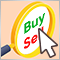构建自动运行的 EA（第 04 部分）：手工触发器（I）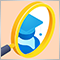构建自动运行的 EA（第 02 部分）：开始编码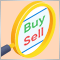构建自动运行的 EA（第 05 部分）：手工触发器（II）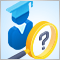构建自动运行的 EA（第 01 部分）：概念和结构